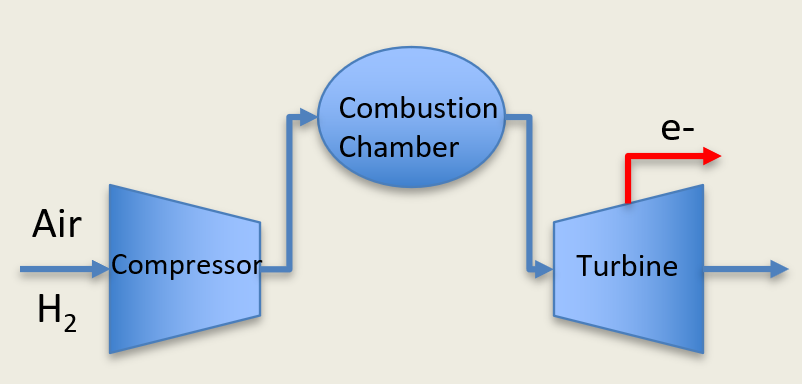# Hydrogen Turbine

The DISPATCHES Hydrogen Turbine Model## Degrees of Freedom

The Hydrogen Turbine Model has 13 degrees of freedom.

## Model Structure

The Hydrogen Turbine Model consists of a Compressor –> Stoichiometric Reactor –> Turbine. The Hydrogen is assumed to be compressed alongside the air that is flowing into the compressor rather than having a separate fuel injection system.

## Variables Used

The Hydrogen Turbine Model uses the follow variables:

Variable

Name

Notes

$$m_{flow}$$

compressor.inlet.flow_mol

Total Molar flow rate into the inlet of the Compressor

$$T_{inlet}$$

compressor.inlet.temperature

Inlet Temperature of stream into the Compressor of the hydrogen turbine

$$P_{inlet}$$

compressor.inlet.pressure

Inlet Pressure into the Compressor

$$w_{Oxygen}$$

compressor.inlet.mole_frac_comp[0, “oxygen”]

Mole fraction of oxygen entering the Compressor Inlet

$$w_{Argon}$$

compressor.inlet.mole_frac_comp[0, “argon”]

Mole fraction of argon entering the Compressor Inlet

$$w_{Nitrogen}$$

compressor.inlet.mole_frac_comp[0, “nitrogen”]

Mole fraction of nitrogen entering the Compressor Inlet

$$w_{water}$$

compressor.inlet.mole_frac_comp[0, “water”]

Mole fraction of water entering the Compressor Inlet

$$w_{Hydrogen}$$

compressor.inlet.mole_frac_comp[0, “hydrogen”]

Mole fraction of hydrogen entering the Compressor Inlet

$$dP_{comp}$$

compressor.deltaP

Pressure change across the compressor

$$\eta_{comp}$$

compressor.efficiency_isentropic

Compressor isentropic efficiency Value

$$\epsilon_{RX}$$

stoic_reactor.conversion

Conversion Rate inside the stoichiometric reactor. Value between [0,1]

$$dP_{turb}$$

turbine.deltaP

Pressure change across the turbine

$$\eta_{turb}$$

turbine.efficiency_isentropic

Turbine isentropic efficiency Value

## Hydrogen Turbine Costs

Hydrogen Turbines are a cutting edge technology that looks to modify the years of work on natural gas turbines to burn hydrogen as opposed to natural gas. As such, baseline costs of natural gas turbines with a modifier placed on top of them is considered.

Natural Gas Capital Costs

Value

Capital Costs:

$947/kW -$1061/kW 

Fixed OPEX:

$7-16.30/kw-yr  Variable OPEX:$4.25/MWh - \$4.29/MWh 

Scaling Factor:

0.72 - 0.78 [0.72 if the entire plant is scaled, 0.78 if only the turbine is scaled] 

References . Rabiti, C., Epiney, A., Talbot, P., Kim, J. S., Bragg-Sitton, S., Alfonsi, A., Yigitoglu, A., Greenwood, S., Cetiner, S. M., Ganda, F., and Maronati, G. Status Report on Modelling and Simulation Capabilities for Nuclear-Renewable Hybrid Energy Systems. United States: N. p., 2017. Web. doi:10.2172/1408526. . Capital Cost and Performance Charasteristic Estimates for Utility Scale Electric Power Generating Technologies. U.S. Energy Information Administration (EIA). February 2020.

## Hydrogen Turbine

class dispatches.unit_models.hydrogen_turbine_unit.HydrogenTurbine(*args, **kwds)

Simple 0D hydrogen turbine unit model

Parameters
• rule (function) – A rule function or None. Default rule calls build().

• concrete (bool) – If True, make this a toplevel model. Default - False.

• ctype (class) –

Pyomo ctype of the block. Default - pyomo.environ.Block

Config args
dynamic

HydrogenTurbine does not support dynamic models, thus this must be False.

has_holdup

HydrogenTurbine does not support dynamic models, thus this must be False.

property_package

Property parameter object used to define property calculations, default - useDefault. Valid values: { useDefault - use default package from parent model or flowsheet, PropertyParameterObject - a PropertyParameterBlock object.}

property_package_args

A ConfigBlock with arguments to be passed to a property block(s) and used when constructing these, default - None. Valid values: { see property package for documentation.}

reaction_package

Reaction parameter object used to define reaction calculations, default - None. Valid values: { None - no reaction package, ReactionParameterBlock - a ReactionParameterBlock object.}

reaction_package_args

A ConfigBlock with arguments to be passed to a reaction block(s) and used when constructing these, default - None. Valid values: { see reaction package for documentation.}

• initialize (dict) – ProcessBlockData config for individual elements. Keys are BlockData indexes and values are dictionaries with config arguments as keys.

• idx_map (function) – Function to take the index of a BlockData element and return the index in the initialize dict from which to read arguments. This can be provided to override the default behavior of matching the BlockData index exactly to the index in initialize.

Returns

(HydrogenTurbine) New instance